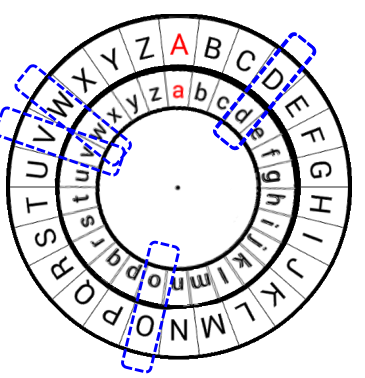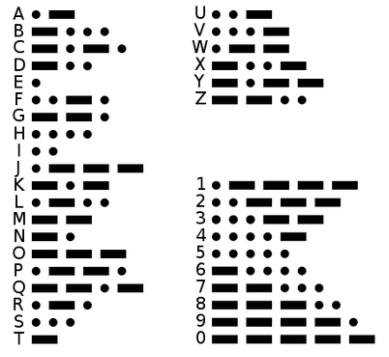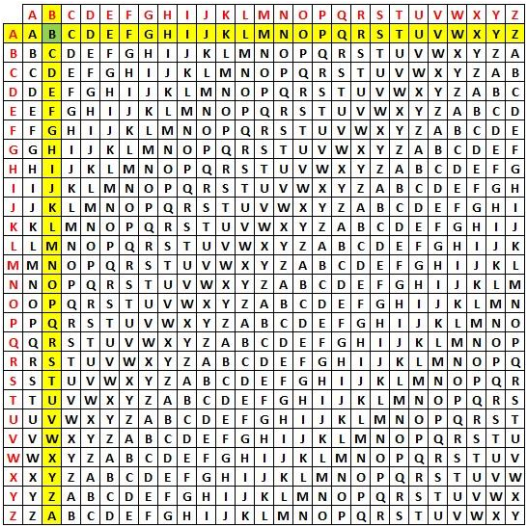# 古典密码学概述

## 2. 替换密码 Substitution cipher

• 替换密码依赖与固定的替换结构
• 对于字母表中的每一个字母的替换都是固定的

【注】

1. 一次替换一个字符显然会在密文中留下太多的明文结构
2. 如果已知明文的性质/结构，则可以通过统计攻击轻松破解任何替换密码

### 2.1 单字母单表密码 Monoalphabetic cipher

• 凯撒密码 Caesar cipher
1. 密钥 $k \in \{0,1,\cdots,25\}$，字母表 $\{a,b,\cdots,z\}$ 与集合 $\{0,1,\cdots,25\}$ 对应。
• 明文空间 = 密文空间 = $\Sigma^+$（其中 $\Sigma$ 为字母表 $\{a,b,\cdots,z\}$
• 密钥空间 = $26$
1. 设密钥为 $k = 3$，明文为 $m = are \ you \ ready$，密文为 $c$。则：
• 加密

$\begin{array}{c} c_i = Enc(m_i,k) = (m_i+3) \,\, mod \,\, 26 \end{array}$

$\begin{array}{c} c = DUH \ BRX \ UHDGB \end{array}$

• 解密

$\begin{array}{c} m_i = Dec(c_i,k) = (c_i-3) \,\, mod \,\, 26 \end{array}$

$\begin{array}{c} m = are \ you \ ready \end{array}$• 原理：令 $p_i$ 指示在正常的英文内容中第 $i$ 个字符出现的频率。则有统计公式：

$\begin{array}{c} \sum_{i=0}^{25} p_i^2 \approx 0.065 \end{array}$

• 方法：
1. 定义

$\begin{array}{c} I_j = \sum_{i=0}^{25} p_i \cdot q_{i+j} \end{array}$

1. 计算 $j \in \{0,1,\cdots,25\}$ 对应的 $I_j$ 的值。
2. 选择 $I_j$ 值接近 $0.065$$j$ 值输出，这些 $j$ 值很可能是密钥 $k$
3. 对于每一个可能的 $j$ 值，计算解密后的原文，看是否有实际意义，有则说明该 $j$ 值即密钥，无则说明不是。
• Mixed alphabetic cipher
字母表 $\{a,b,\cdots,z\}$ 到字母表 $\{A,B,\cdots,Z\}$ 的映射是一个置换，每个小写字母（代表明文）分别映射到一个唯一的大写字母（表示密文）。
1. 密钥空间 = $26!$
2. 每个字母的映射是固定的
3. 已知语言中单个字母的概率分布
• 摩斯码 Morse code
每个字母映射为一系列点和短横线。

1. 一条短横线等于三个点。
2. 一个字母对应的系列点和短横线间的空格间隔等于一个点长度
3. 两个相邻字母间的空格间隔等于三个点的长度
4. 两个单词间的空格间隔等于七个点的长度### 2.2 单字母多表密码 Polyalphabetic cipher

• 维吉尼亚密码
1. 给定一定长度密钥，重复密钥直至密钥流和明文长度相同。
2. 加密

$\begin{array}{c} c_i = (m_i + k_{i \,\, mod \,\, l}) \,\, mod \,\, 26 \end{array}$

1. 解密

$\begin{array}{c} m_i = (c_i - k_{i \ mod \ l}) \ mod \ 26 \end{array}$

1. 根据加解密公式可以构造出表格法
假如明文为 $a simple example$，密钥为 $battista$，则表格法加密过程为：
• 生成密钥流与明文字符一一对应：
Plaintext a s i m p l e e x a m p l e
Keystream b a t t i s t a b a t t i s
• 参照表格（Tabula recta）计算密文。其中，明文字符对应行索引，密钥字符对应列索引：• 最终计算得到的密文为：
Plaintext a s i m p l e e x a m p l e
Keystream b a t t i s t a b a t t i s
Ciphertext B S B F X D X E Y A F I T W
• 解密过程就是加密的逆过程。根据密钥字符对应的列，寻找密文字符，则密文字符在表格中对应的行索引字符即明文字符。
OTP 是唯一一个达到完美加密的加密系统，无法被攻破。

1. OTP 的安全性完全取决于密钥的随机性，即密钥必须是随机产生的。
2. 密钥长度必须大于等于明文长度。
3. 密钥只能使用一次，不能重复使用。
4. 密钥必须完全保密。

1. 将字母表 $\{a,b,\cdots,z\}$ 映射到数字集合 $\{0,1,\cdots,25\}$。得到：
• This is an example → 19 7 8 18 8 18 0 13 4 23 0 12 15 11 4
• MASKL NSFLD FKJPQ → 12 0 18 10 11 13 18 5 11 3 5 10 9 15 16
1. 二者依序相加并模 26 得到密文消息如下：
• 5 7 0 2 19 5 18 18 15 0 5 22 24 0 20 → FHACTFSSPAFWYAU
1. 解密即反向操作即可。

### 2.3 多字母单表密码 Multiple letter cipher

• 波雷费密码 Playfair cipher
Playfair 密码是首种双字母替换密码。

1. 选取一个 keyword 作为密钥，去除密钥中重复出现的字母，将密钥的字母逐个从左到右，从上到下加入 $5 \times 5$ 的矩阵中，剩下的空间将未加入的英文字母依照 $a-z$ 的顺序加入，将字母将 $I$$J$ 视为同一字符（或将 $Q$ 去除）。
2. 将要加密的明文分成两个一组。若组内的字母相同，将X（或Q）插入两字母之间，重新分组（例如 HELLO 将分成 HE LX LO）。若剩下一个字，也加入X字。
3. 在每组中，找出两个字母在矩阵中的地方。
• 若两个字母不在同一直行或同一横列，在矩阵中找出另外两个字母，使这四个字母成为一个长方形的四个角（读取按行对应，即两个字母分别依次对应同行的那个字母）
• 若两个字母在同一横行，取这两个字母右方的字母（若字母在最右方则取最左方的字母）。
• 若两个字母在同一直列，取这两个字母下方的字母（若字母在最下方则取最上方的字母）。
1. 新找到的两个字母就是原本的两个字母加密的结果。
• 希尔密码 Hill cipher
希尔密码是运用基本矩阵论原理的替换密码，一次性替换三字母。

1. 将字母表 $\{a,b,\cdots,z\}$ 映射到数字集合 $\{0,1,\cdots,25\}$
2. 加密密钥是一个 $3 \times 3$ 的可逆矩阵（如果不可逆则无法解密）：

$\begin{array}{c} K = \left[ \begin{matrix} k_{11} & k_{12} & k_{13} \\ k_{21} & k_{22} & k_{23} \\ k_{31} & k_{31} & k_{33} \\ \end{matrix} \right] \end{array}$

1. 明文被排列为以下格式：

$\begin{array}{c} M = \left[ \begin{matrix} m_1 \\ m_2 \\ m_3 \\ \end{matrix} \right] \end{array}$

1. 加密公式为：

$\begin{array}{c} C = \left[ \begin{matrix} c_1 \\ c_2 \\ c_3 \\ \end{matrix} \right] = (K \cdot M) \ mod \ 26 = (\left[ \begin{matrix} k_{11} & k_{12} & k_{13} \\ k_{21} & k_{22} & k_{23} \\ k_{31} & k_{31} & k_{33} \\ \end{matrix} \right] \cdot \left[ \begin{matrix} m_1 \\ m_2 \\ m_3 \\ \end{matrix} \right]) \ mod \ 26 \end{array}$

1. 解密公式为：

$\begin{array}{c} M = (K^{-1}C) \ mod \ 26 = (K^{-1}KM) \ mod \ 26 = M \end{array}$

## 3. 置换密码 Transposition cipher

• 篱笆密码法 Rail Fence cipher
明文由上至下顺序写上，当到达最低部时，再回头向上，一直重复直至整篇明文写完为止。然后，再往右顺序抄写一次，即得到密文。

1. 明文：WE ARE DISCOVERED. FLEE AT ONCE
2. 篱笆密码法：
1. 密文：WECRL TEERD SOEEF EAOCA IVDEN
• Column Transposition cipher
将明文按行顺序排列，超过行长则另起一行。密钥为一个置换，密钥长度决定行的长度。根据密钥指定的置换顺序，一列一列读取字符组在一起得到密文。

1. 密钥：4 3 1 2 5 6 7
2. 明文：attack postponed until two am
3. 置换：
4 3 1 2 5 6 7
a t t a c k p
o s t p o n e
d u n t i l t
w o a m x y z
1. 密文：TTNAAPTMTSUOAODWCOIXKNLYPETZ

## 4. 转轮密码机 Rotor machine

• German Enigma
• Allied Hagelin
• Japanese Purple